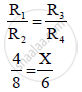HSC Science (Electronics) 12th Board ExamMaharashtra State Board
Share

# Four Resistances 4ω,8ω,Xω, and 6ω Are Connected in a Series So as to Form Wheatstone’S Network. If the Network is Balanced, Find the Value of ‘X - HSC Science (Electronics) 12th Board Exam - Physics

#### Question

Four resistances 4Ω,8Ω,XΩ, and 6Ω are connected in a series so as to form Wheatstone’s
network. If the network is balanced, find the value of ‘X’.

#### Solution

R1 = 4Ω, R2 = 8Ω, R3 = XΩ, R4 = 6Ω

When Wheatstone’s network is balanced,∴ X = 3 Ω

The value of ‘X’ is 3Ω.

Is there an error in this question or solution?

#### APPEARS IN

2012-2013 (October) (with solutions)
Question 7.6 | 2.00 marks

#### Video TutorialsVIEW ALL 

Solution Four Resistances 4ω,8ω,Xω, and 6ω Are Connected in a Series So as to Form Wheatstone’S Network. If the Network is Balanced, Find the Value of ‘X Concept: Wheatstone Bridge.
S